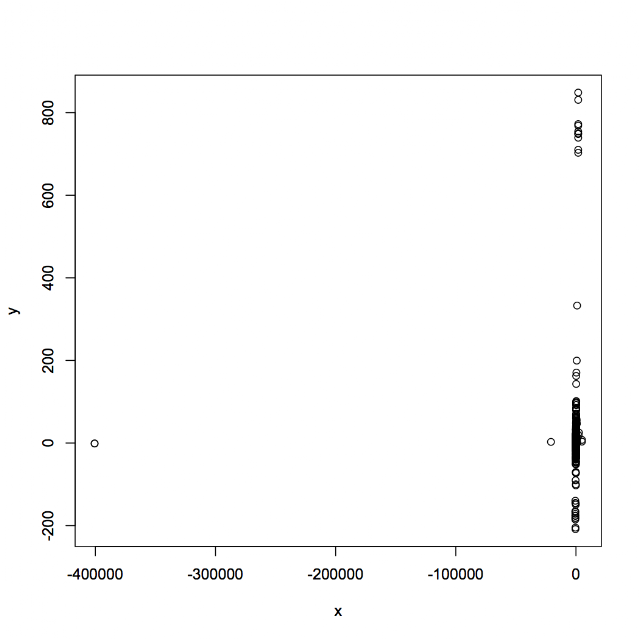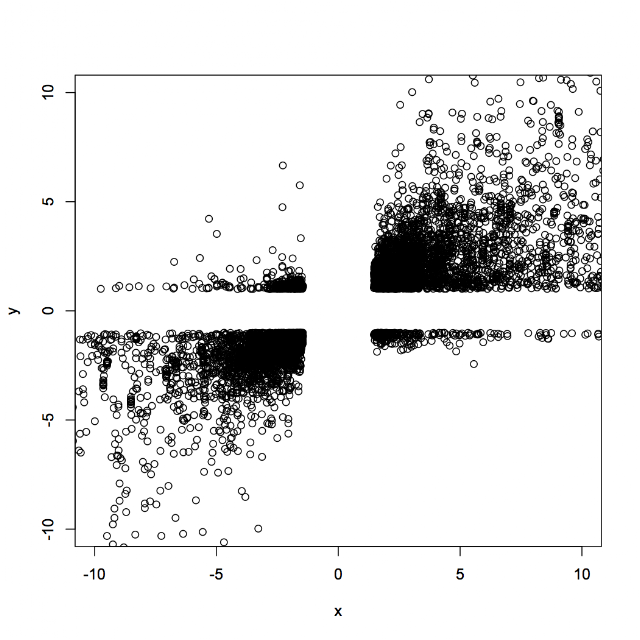## Monday, November 26, 2012

### How to set limits to a graph in R using plot

If you use plot command to do a scatterplot between two variables in R and if you have one variable containing a numerical value that is way off limits the scatterplot will consider a complete range of that variable (including the one off limit) and will not represent the relationship between variables in a good manner.

For example x and y are variables with 9986 numerical values.

To do a scatterplot type:

plot(x,y)

What you get is a graph that contains one outlier that is messing up the whole graph (that particular point contains the value for x of ~ -400000 and it completely masks the entire relationship between x and y).Type:

plot(x,y, ylim=c(-10,10), xlim=c(-10,10))

to limit the x and y axes to -10 to 10 regions.